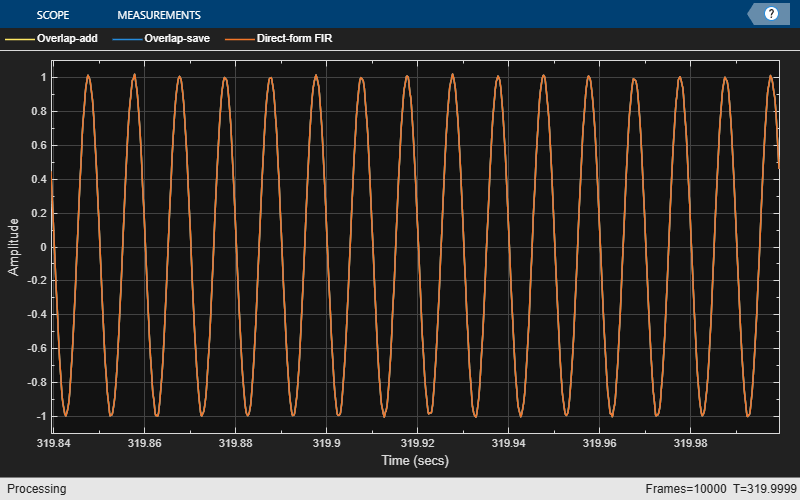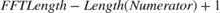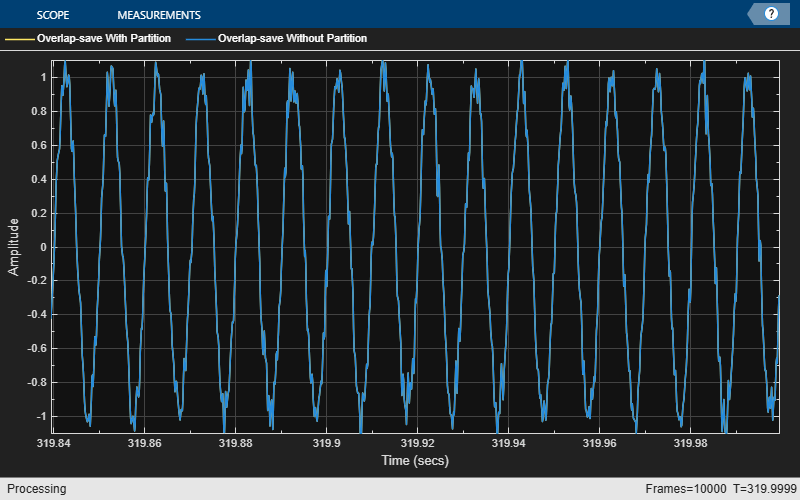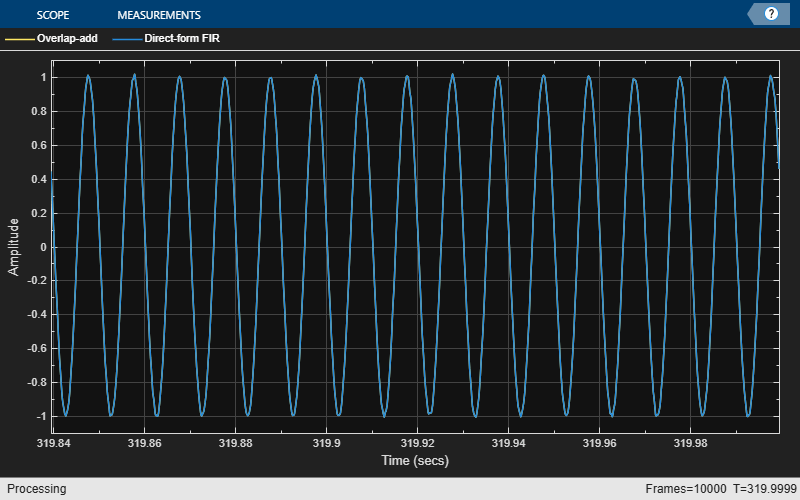dsp.FrequencyDomainFIRFilter

Filter input signal in frequency domain

Description

The dsp.FrequencyDomainFIRFilter System object™ implements frequency-domain, fast Fourier transform (FFT)-based filtering to filter a streaming input signal. In the time domain, the filtering operation involves a convolution between the input and the impulse response of the finite impulse response (FIR) filter. In the frequency domain, the filtering operation involves the multiplication of the Fourier transform of the input and the Fourier transform of the impulse response. The frequency-domain filtering is efficient when the impulse response is very long. You can specify the filter coefficients directly in the frequency domain by setting NumeratorDomain to 'Frequency'.

This object uses the overlap-save and overlap-add methods to perform the frequency-domain filtering. For filters with a long impulse response length, the latency inherent to these two methods can be significant. To mitigate this latency, the dsp.FrequencyDomainFIRFilter object partitions the impulse response into shorter blocks and implements the overlap-save and overlap-add methods on these shorter blocks. To partition the impulse response, set the PartitionForReducedLatency property to true. For more details on these two methods and on reducing latency through impulse response partitioning, see Algorithms.

To filter the input signal in the frequency domain:

1. Create the dsp.FrequencyDomainFIRFilter object and set its properties.

2. Call the object with arguments, as if it were a function.

Creation

Description

fdf = dsp.FrequencyDomainFIRFilter creates a frequency domain FIR filter System object that filters each channel of the input signal independently over time in the frequency domain using the overlap-save or overlap-add method.

example

fdf = dsp.FrequencyDomainFIRFilter(num) creates a frequency domain FIR filter object with the Numerator property set to num.

Example: dsp.FrequencyDomainFIRFilter(fir1(400,2 * 2000 / 8000));

example

fdf = dsp.FrequencyDomainFIRFilter(Name,Value) creates a frequency domain FIR filter System object with each specified property set to the specified value. Enclose each property name in single quotes. You can use this syntax with any previous input argument combinations.

Properties

expand all

Unless otherwise indicated, properties are nontunable, which means you cannot change their values after calling the object. Objects lock when you call them, and the release function unlocks them.

If a property is tunable, you can change its value at any time.

Frequency-domain filter method, specified as either 'Overlap-save' or 'Overlap-add'. For more details on these two methods, see Algorithms.

Domain of the filter coefficients, specified as one of the following:

• 'Time' –– Specify the time-domain filter numerator in the Numerator property.

• 'Frequency' –– Specify the filter's frequency response in the FrequencyResponse property.

FIR filter coefficients, specified as a row vector.

Tunable: Yes

Dependencies

This property applies when NumeratorDomain is set to 'Time'.

Data Types: single | double | int8 | int16 | int32 | int64 | uint8 | uint16 | uint32 | uint64
Complex Number Support: Yes

Frequency response of the filter, specified as a row vector or a matrix. When PartitionForReducedLatency is false, FrequencyResponse must be a row vector. The FFT length is equal to the length of the FrequencyResponse vector. When PartitionForReducedLatency is true, FrequencyResponse must be a 2P-by-N matrix, where P is the partition size, and N is the number of partitions.

Tunable: Yes

Dependencies

This property applies when NumeratorDomain is set to 'Frequency'.

Data Types: single | double | int8 | int16 | int32 | int64 | uint8 | uint16 | uint32 | uint64
Complex Number Support: Yes

Time-domain numerator length, specified as a positive integer-valued scalar.

Dependencies

This property applies when NumeratorDomain is set to 'Frequency' and PartitionForReducedLatency is set to false.

Data Types: single | double | int8 | int16 | int32 | int64 | uint8 | uint16 | uint32 | uint64

Flag to specify if the filter coefficients are all real, specified as true or false.

Dependencies

This property applies when NumeratorDomain is set to 'Frequency'.

Flag to partition numerator to reduce latency, specified as one of the following:

• false –– The filter uses the traditional overlap-save or overlap-add method. The latency in this case is FFTLength – length(Numerator) + 1.

• true –– In this mode, the object partitions the numerator into segments of length specified by the PartitionLength property. The filter performs overlap-save or overlap-add on each partition, and combines the partial results to form the overall output. The latency is now reduced to the partition length.

FFT length, specified as a positive integer. The default value of this property, [], indicates that the FFT length is equal to twice the numerator length. The FFT length must be greater than or equal to the numerator length.

Example: 64

Dependencies

This property applies when you set NumeratorDomain property to 'Time' and PartitionForReducedLatency property to false.

Data Types: single | double | int8 | int16 | int32 | int64 | uint8 | uint16 | uint32 | uint64

Numerator partition length, specified as a positive integer less than or equal to the length of the numerator.

Example: 40

Example: 60

Dependencies

This property applies when you set the NumeratorDomain property to 'Time' and PartitionForReducedLatency property to true.

Data Types: single | double | int8 | int16 | int32 | int64 | uint8 | uint16 | uint32 | uint64

Filter latency in samples, returned as an integer greater than 0. When PartitionForReducedLatency is false, the latency is equal to FFTLength – length(Numerator) + 1. When PartitionForReducedLatency is true, the latency is equal to the partition length.

Data Types: uint32

Usage

Description

example

fdfOut = fdf(input) filters the input signal and outputs the filtered signal. The object filters each channel of the input signal independently over time in the frequency domain.

Input Arguments

expand all

Data input, specified as a vector or a matrix. This object supports variable-size input signals. That is, you can change the input frame size (number of rows) even after calling the algorithm. However, the number of channels (number of columns) must remain constant.

Example: randn(164,4)

Data Types: single | double
Complex Number Support: Yes

Output Arguments

expand all

Filtered output, returned as a vector or matrix. The size, data type, and complexity of the output match those of the input.

Data Types: single | double
Complex Number Support: Yes

Object Functions

To use an object function, specify the System object as the first input argument. For example, to release system resources of a System object named obj, use this syntax:

release(obj)

expand all

 fvtool Visualize frequency response of DSP filters
 step Run System object algorithm release Release resources and allow changes to System object property values and input characteristics reset Reset internal states of System object

Examples

collapse all

Filter input signal using overlap-add and overlap-save methods, and compare the outputs to the output of a FIR filter.

Initialization

Design the FIR lowpass filter coefficients using the fir1 function. The sampling frequency is 8 kHz, and the cutoff frequency of the filter is 2 kHz. The impulse response has a length of 400.

impL    = 400;
Fs      = 8000;
Fcutoff = 2000;
imp     = fir1(impL,2*Fcutoff/Fs);

Create two dsp.FrequencyDomainFIRFilter objects and a dsp.FIRFilter object. Set the numerator of all the three filters to imp. Delay the FIR output by the latency of the frequency-domain filter.

fdfOS = dsp.FrequencyDomainFIRFilter(imp,'Method','overlap-save');
fir = dsp.FIRFilter('Numerator',imp);
dly = dsp.Delay('Length',fdfOA.Latency);

Create two dsp.SineWave objects. The sine waves generated have a sample rate of 8000 Hz, frame size of 256, and frequencies of 100 Hz and 3 kHz, respectively. Create a timescope object to view the filtered outputs.

frameLen  = 256;

sin_100Hz = dsp.SineWave('Frequency',100,'SampleRate',Fs,...
'SamplesPerFrame',frameLen);
sin_3KHz  = dsp.SineWave('Frequency',3e3,'SampleRate',Fs,...
'SamplesPerFrame',frameLen);
ts = timescope('TimeSpanOverrunAction','Scroll',...
'ShowGrid',true,'TimeSpanSource','Property','TimeSpan',5 * frameLen/Fs,...
'YLimits',[-1.1 1.1],...
'ShowLegend',true,...
'SampleRate',Fs,...

Streaming

Stream 1e4 frames of noisy input data. Pass this data through the frequency domain filters and the FIR filter. View the filtered outputs in the time scope.

numFrames = 1e4;
for idx = 1:numFrames
x = sin_100Hz() + sin_3KHz() + 0.01*randn(frameLen,1);
yOA = fdfOA(x);
yOS = fdfOS(x);
yFIR = fir(dly(x));
ts([yOA,yOS,yFIR]);
endThe outputs of all the three filters match exactly.

Partition the impulse response length of a frequency domain FIR filter. Compare the outputs of the partitioned filter and the original filter.

Design the FIR lowpass filter coefficients using the fir1 function. The sampling frequency is 8 kHz and the cutoff frequency of the filter is 2 kHz. The impulse response is of length 4000.

impL    = 4000;
Fs      = 8000;
Fcutoff = 2000;
imp     = fir1(impL,2 * Fcutoff / Fs);

Create a dsp.FrequencyDomainFIRFilter with coefficients set to the imp vector. The latency of this filter is given by, which is equal to 4002. By default, FFT length is equal to twice the numerator length. This makes the latency proportional to the impulse response length.

fdfOS  = dsp.FrequencyDomainFIRFilter(imp,'Method','overlap-save');
fprintf('Frequency domain filter latency is %d samples\n',fdfOS.Latency);
Frequency domain filter latency is 4002 samples

Partition the impulse response to blocks of length 256. The latency after partitioning is proportional to the block length.

fdfRL  = dsp.FrequencyDomainFIRFilter(imp,'Method','overlap-save',...
'PartitionForReducedLatency',true,...
'PartitionLength',256);
fprintf('Frequency domain filter latency is %d samples\n',fdfRL.Latency);
Frequency domain filter latency is 256 samples

Compare the outputs of the two frequency domain filters. The latency of fdfOS is 4002, and the latency of fdfRL is 256. To compare the two outputs, delay the input to fdfRL by 4002 - 256 samples.

dly = dsp.Delay('Length',(fdfOS.Latency-fdfRL.Latency));

Create two dsp.SineWave objects. The sine waves have a sample rate of 8000 Hz, frame size of 256, and frequencies of 100 Hz and 3 kHz, respectively. Create a timescope object to view the filtered outputs.

frameLen  = 256;
sin_100Hz = dsp.SineWave('Frequency',100,'SampleRate',Fs,...
'SamplesPerFrame',frameLen);
sin_3KHz  = dsp.SineWave('Frequency',3e3,'SampleRate',Fs,...
'SamplesPerFrame',frameLen);
ts = timescope('TimeSpanOverrunAction','Scroll','ShowGrid',true,...
'TimeSpanSource','Property','TimeSpan',5 * frameLen/Fs,...
'YLimits',[-1.1 1.1],...
'ShowLegend',true,...
'SampleRate',Fs,...
'ChannelNames',{'Overlap-save With Partition','Overlap-save Without Partition'});

Stream 1e4 frames of noisy input data. Pass this data through the two frequency domain filters. View the filtered outputs in the time scope.

numFrames = 1e4;
for idx = 1:numFrames
x = sin_100Hz() + sin_3KHz() + .1 * randn(frameLen,1);
yRL = fdfRL(dly(x));
yOS = fdfOS(x);
ts([yRL,yOS]);
endThe outputs match exactly.

Specify the numerator coefficients of the frequency-domain FIR filter in the frequency domain. Filter the input signal using the overlap-add method. Compare the frequency-domain FIR filter output to the corresponding time-domain FIR filter output.

Initialization

Design the FIR lowpass filter coefficients using the fir1 function. The sampling frequency is 8 kHz, and the cutoff frequency of the filter is 2 kHz. The time-domain impulse response has a length of 400. Compute the FFT of this impulse response and specify this response as the frequency response of the frequency-domain FIR filter. Set the time-domain numerator length, specified by the NumeratorLength property, to the number of elements in the time-domain impulse response.

impL    = 400;
Fs      = 8000;
Fcutoff = 2000;
imp     = fir1(impL,2 * Fcutoff / Fs);
H   = fft(imp , 2 * numel(imp));
oa  = dsp.FrequencyDomainFIRFilter('NumeratorDomain','Frequency',...
'FrequencyResponse', H,...
'NumeratorLength',numel(imp),...
fprintf('Frequency domain filter latency is %d samples\n',oa.Latency);
Frequency domain filter latency is 402 samples

Create a dsp.FIRFilter System object? and specify the numerator as the time-domain coefficients computed using the fir1 function, imp. Delay the FIR output by the latency of the frequency-domain FIR filter.

fir = dsp.FIRFilter('Numerator',imp);
dly = dsp.Delay('Length',oa.Latency);

Create two dsp.SineWave objects. The sine waves generated have a sample rate of 8000 Hz, frame size of 256, and frequencies of 100 Hz and 3 kHz, respectively. Create a timescope object to view the filtered outputs.

frameLen  = 256;

sin_100Hz = dsp.SineWave('Frequency',100,'SampleRate',Fs,...
'SamplesPerFrame',frameLen);
sin_3KHz  = dsp.SineWave('Frequency',3e3,'SampleRate',Fs,...
'SamplesPerFrame',frameLen);

ts = timescope('TimeSpanOverrunAction','Scroll',...
'ShowGrid',true,'YLimits',[-1.1 1.1],...
'TimeSpanSource','Property','TimeSpan',5 * frameLen/Fs,...
'ShowLegend',true,...
'SampleRate',Fs,...

Streaming

Stream 1e4 frames of noisy input data. Pass this data through the frequency-domain FIR filter and the time-domain FIR filter. View the filtered outputs in the time scope.

numFrames = 1e4;
for idx = 1:numFrames
x = sin_100Hz() + sin_3KHz() + 0.01 * randn(frameLen,1);
y1 = oa(x);
y2 = fir(dly(x));
ts([y1,y2]);
endThe outputs of both the filters match exactly.

Algorithms

expand all

Overlap-save and overlap-add are the two frequency-domain FFT-based filtering methods this algorithm uses.

 Stockham, T. G., Jr. "High Speed Convolution and Correlation." Proceedings of the 1966 Spring Joint Computer Conference, AFIPS, Vol 28, 1966, pp. 229–233.

DSP System Toolbox Documentation평가판 신청